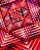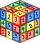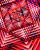# GP members

The geometric sequence has 10 members. The last two members are 2 and -1. Which member is -1/16?

x =  14

### Step-by-step explanation:Did you find an error or inaccuracy? Feel free to write us. Thank you!## Related math problems and questions:

• Geometric sequence 5About members of geometric sequence we know: ? ? Calculate a1 (first member) and q (common ratio or q-coefficient)
• Five elementThe geometric sequence is given by quotient q = 1/2 and the sum of the first six members S6 = 63. Find the fifth element a5.
• Geometric seqFind the third member of geometric progression if a1 + a2 = 36 and a1 + a3 = 90. Calculate its quotient.
• Sequences AP + GPThe three numbers that make up the arithmetic sequence have the sum of 30. If we subtract from the first 5, from the second 4 and keep the third, we get the geometric sequence. Find AP and GP members.
• Geometric progressionIn geometric progression, a1 = 7, q = 5. Find the condition for n to sum first n members is sn≤217.
• Geometric sequence 4It is given geometric sequence a3 = 7 and a12 = 3. Calculate s23 (= sum of the first 23 members of the sequence).
• Fifth memberDetermine the fifth member of the arithmetic progression, if the sum of the second and fifth members equal to 73, and difference d = 7.
• GP - three membersThe second and third of a geometric progression are 24 and 12(c+1), respectively, given that the sum of the first three terms of progression is 76. determine the value of c.
• Five membersWrite first 5 members geometric sequence and determine whether it is increasing or decreasing: a1 = 3 q = -2
• Tenth memberCalculate the tenth member of geometric sequence when given: a1=1/2 and q=2
• QuotientDetermine the quotient and the second member of the geometric progression where a3=10, a1+a2=-1,6 a1-a2=2,4.
• Geometric sequenceIn the geometric sequence is a4 = 20 a9 = -160. Calculate the first member a1 and quotient q.
• Sequence - 5 membersWrite first five members of the sequence ?
• GP - sequenceThe first three terms of a geometric sequence are as follows 10, 30. 90, find the next two terms of this sequence.
• HP - harmonic progression 2Compute the 16th term of the HP if the 6th and 11th term of the harmonic progression are 10 and 18 respectively.
• Geometric sequence 3In geometric sequence is a8 = 312500; a11= 39062500; sn=1953124. Calculate the first item a1, quotient q, and n - number of members by their sum s_n.
• SequenceCalculate what member of the sequence specified by (5n-2+15) has value 20.